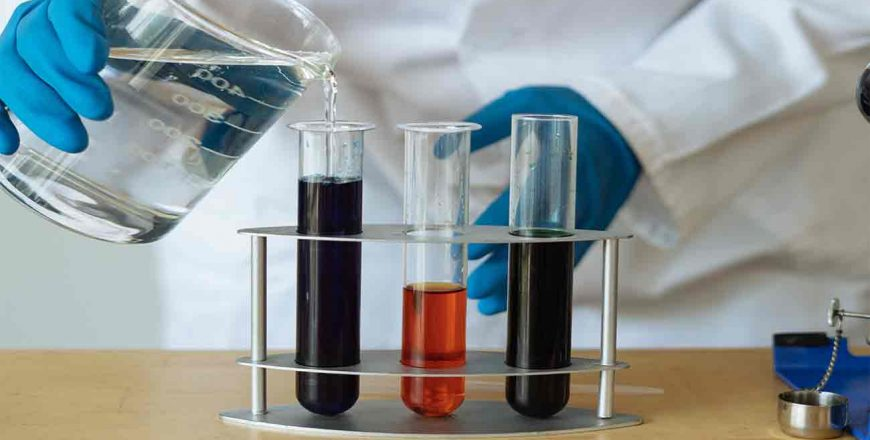# Chapter 4 Stoichiometry Module 1 Weekly Class

Category:Course Structure
Module 1 of Chapter 4 Stoichiometry you will learn

• Stoichiometry
• The mole concept

Course Information

4 x Regular weekly live online lesson
Instant Q&A with teacher during online lessons

Course Syllabus

Chapter 4 – Stoichiometry
Cambridge IGCSE 0620
For examination in June and November 2020 and 2021

Teachers & Curriculum Expert

Course prepared by
Mr Sai Mun
Mr Khing

Click curriculum tab on top to learn now

### 4.1 Stoichiometry

1
Relative Atomic Mass (Ar)
2
Relative Molecular Mass (Mr)
3
Relative Formula Mass (Mr)
4
Chemical Formulae
5
Question 1 – Formula of compund
6
Question 2 – Compunds and their chemical formula
7
Chemical Equations
8
Question 1 – Balance the equation
9
Question 2 – Balanced symbol equation

### 4.2 The mole concept

1
The Mole Concept
2
Question 1 – Mass of One Mole
3
Question 2 – Mass of moles
4
Reacting Masses **
5
Question 1 – Mass of Magnesium Oxide
6
Question 2 – Mass of Cooper (II) Sulfate
7
Question 3 – Number of Moles & Mass of Barium Oxide
8
Question 4 – Number of Moles of Oxygen Molecules
9
Question 5 – Volumes of Oxygen
10
Reacting Volumes **
11
Question 1 – Volume of Oxygen Needed
12
Question 2 – Volume of Oxygen Needed
13
Question 3 – Volume of Sulfur Trioxide Formed
14
Question 4 – Volume of Carbon Dioxide Produced
15
Question 5 – Mass of Aluminium Used
16
Concentration of Solutions and Number of Moles **
17
Question 1 – Concentration of Solutions
18
Question 2 – Mass of Solute Needed
19
Question 3 – Concentration of Acid
20
Percentage of Yield
21
Question 1 – Percentage of Yield
22
Question 2 – Percentage of Yield
23
Question 3 – Percentage of Yield
24
Percentage of Purity
25
Question 1 – Percentage Purity of Calcium Carbonate
26
Question 2 – Percentage Purity of Sodium Hydrogencarbonate
27
Question 3 – Limiting Reagent
28
Empirical Formula
29
Determine the Empirical Formula
30
Question 1 – Determine The Empirical Formula
31
Question 2 – Empirical Formula of Calcium Carbonate
32
Question 3 – Empirical Formula of Aluminium Oxide
33
Question 4 – Empirical Formula of Compound
34
Question 5 – Molecular Formula of Hydrocarbon
35
Question 6 – Calculate The Empirical Formula

1
Quiz 1
20 questions
2
Quiz 2
20 questions
3
Quiz 3
20 questions
4
Quiz 4
20 questions
5
Quiz 5
20 questions